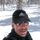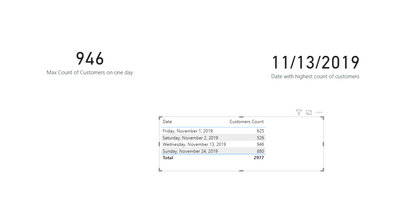cancel
Showing results for
Did you mean:Regular Visitor

## DAX measure to show the max customers count and the corresponding date by grouping of date field

Hello All,

I have a requirement to create a DAX measure to calculate to show the max customers count by grouping them on date and to display the corresponding day with max customers count.  I am using directy query and not storing any data inside Power BI

Below is the dummied data table to illustrate my scenario.Here, i wanted to calcualate the sum of customers count by grouping them on Date column, then display the date with maxium customers count and also want to display the max customers count.

I have also provided the table after grouping by date. At the end, i wanted to display the max customers count (946) and the corresponding date (11/13/2019).

I would appreciate any suggestions or feedback to get the max customers count and the corresponding date field using DAX measure in Direct Query.

Fact Table

 Date Customers Count 11/1/2019 230 11/1/2019 150 11/1/2019 245 11/2/2019 165 11/2/2019 176 11/2/2019 185 11/13/2019 56 11/13/2019 320 11/13/2019 120 11/13/2019 450 11/24/2019 190 11/24/2019 120 11/24/2019 340 11/24/2019 230

Table after grouping the data based on date field

 Date Customers Count 11/1/2019 625 11/2/2019 526 11/13/2019 946 11/24/2019 880

Thank you!!

2 ACCEPTED SOLUTIONSSuper User

Hello @manojsv19 ,

Try this:First measure

``````Max Count of Customers on one day =

VAR _newTable =
'Table',
"Sum of customers per d", CALCULATE (
SUM ( 'Table'[Customers Count] ),
ALLEXCEPT ( 'Table', 'Table'[ Date] )
)
)
VAR _newTableMax =
MAXX ( _newTable, [Sum of customers per d] )

RETURN
_newTableMax``````

Created a calculated column

``Sum of customers per date = CALCULATE(sum('Table'[Customers Count]),ALLEXCEPT('Table','Table'[ Date]))``

Last measure

``````Date with highest count of customers =
var _maxCount = MAX('Table'[Sum of customers per date])
return
CALCULATE(MAX('Table'[ Date]),FILTER(ALLEXCEPT('Table','Table'[ Date]),'Table'[Sum of customers per date]=_maxCount))``````

Let me know if you have any questions.

If this solves your issues, please mark it as the solution, so that others can find it easily. Kudos 👍are nice too.
Nathaniel

Proud to be a Super User!Super User

Hi @manojsv19 ,

Figured out the syntax of a the virtual table so that you don't need to create a calculated column. This replaces the last measure.

``````Date with highest count of customers 2 =
VAR _newTable =
'Table',
"Sum of customers per d", CALCULATE (
SUM ( 'Table'[Customers Count] ),
ALLEXCEPT ( 'Table', 'Table'[ Date] )
)
)
VAR _newTableMax =
MAXX ( _newTable, [Sum of customers per d] )

var _calc = CALCULATE(MAX('Table'[ Date]),FILTER(_newTable,MAX([Sum of customers per d] )= _newTableMax))

RETURN
_calc``````

Let me know if you have any questions.

If this solves your issues, please mark it as the solution, so that others can find it easily. Kudos 👍are nice too.
Nathaniel

Proud to be a Super User!

2 REPLIES 2Super User

Hello @manojsv19 ,

Try this:First measure

``````Max Count of Customers on one day =

VAR _newTable =
'Table',
"Sum of customers per d", CALCULATE (
SUM ( 'Table'[Customers Count] ),
ALLEXCEPT ( 'Table', 'Table'[ Date] )
)
)
VAR _newTableMax =
MAXX ( _newTable, [Sum of customers per d] )

RETURN
_newTableMax``````

Created a calculated column

``Sum of customers per date = CALCULATE(sum('Table'[Customers Count]),ALLEXCEPT('Table','Table'[ Date]))``

Last measure

``````Date with highest count of customers =
var _maxCount = MAX('Table'[Sum of customers per date])
return
CALCULATE(MAX('Table'[ Date]),FILTER(ALLEXCEPT('Table','Table'[ Date]),'Table'[Sum of customers per date]=_maxCount))``````

Let me know if you have any questions.

If this solves your issues, please mark it as the solution, so that others can find it easily. Kudos 👍are nice too.
Nathaniel

Proud to be a Super User!Super User

Hi @manojsv19 ,

Figured out the syntax of a the virtual table so that you don't need to create a calculated column. This replaces the last measure.

``````Date with highest count of customers 2 =
VAR _newTable =
'Table',
"Sum of customers per d", CALCULATE (
SUM ( 'Table'[Customers Count] ),
ALLEXCEPT ( 'Table', 'Table'[ Date] )
)
)
VAR _newTableMax =
MAXX ( _newTable, [Sum of customers per d] )

var _calc = CALCULATE(MAX('Table'[ Date]),FILTER(_newTable,MAX([Sum of customers per d] )= _newTableMax))

RETURN
_calc``````

Let me know if you have any questions.

If this solves your issues, please mark it as the solution, so that others can find it easily. Kudos 👍are nice too.
Nathaniel

Proud to be a Super User!